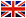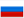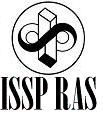Interacting electrons in metals

I.V. Bobkova

1. One-particle Green’s function (Non interacting fermions)
1. Verbal definition of retarded one-particle Green’s function.
2. An example for Green’s function diagram expansion.
2. One-particle Green’s function (Interacting fermions)
1. Formal definition of one-particle Green’s function.
2. Hamiltonian and graphic representation of the interaction between fermions.
3. Quasi particles in Hartree-Fock approximation.
4. Back to quasi particles.
5. Diagrams topology.
3. Dyson equation.
1. Derivation of the Dyson equation.
3. Quasi particles in random phase approximation.
4. Polarization operator in common case.
4. Justification of Fermi liquid.
1. Self consistent perturbation theory.
2. Quasi particles and one-particle Green’s function.
3. Dressed vertex.
5. Ground state energy in random phase approximation.
1. Vacuum amplitude
2. Calculation of the ground state energy.
6. Two particle propagator.
1. Definition of two particle propagator
2. Plasmons.
3. Vertex function and scattering of quasi particles.
4. Excitons.
7. Green’s functions at finite temperature.
1. Formulation of the problem.
2. Perturbation theory for the Matsubara Green;s function.
3. Matsubara Green’s function foe free particles.
8. Green's functions in the theory of superconductivity.
1. Hamiltonian of interacting electrons and phonons.
2. Ground state energy, quasiparticles (based on the BCS method).
3. Nonapplicability of the standard perturbation diagram expansion.
4. Formalism Nambu.

Main references

1. Mattuck R., A guide to Feynman diagrams in the many body problem, McGraw-Hill Publishing Company Limited, 1967.
2. L.S. Levitov and A.V. Shytov, Green's functions. Theory and practice, FizMatLit-Nauka, Moscow, 2003 (in russian).